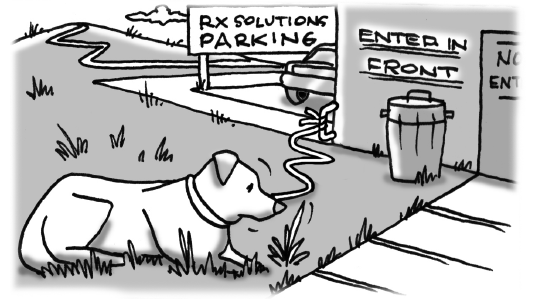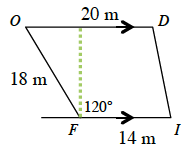### Home > GC > Chapter 9 > Lesson 9.2.2 > Problem9-70

9-70.Find the area of the Marina’s drugstore (FIDO) in problem 9-69. Show all work.

Draw a diagram that will help you solve for the area. Notice the figure being split into smaller more manageable figures, especially right triangles.Find the height of the trapezoid.

Note that the green line that has been drawn has created a special $30°\text{-}60°\text{-}90°$ right triangle. Use this information to solve for $h$.

After solving for $h$, use the formula $\left(\frac{1}{2}\right)\left(b_1+b_2\right)h$ to find the area

$\text{Area}\approx265\text{ m}^2$